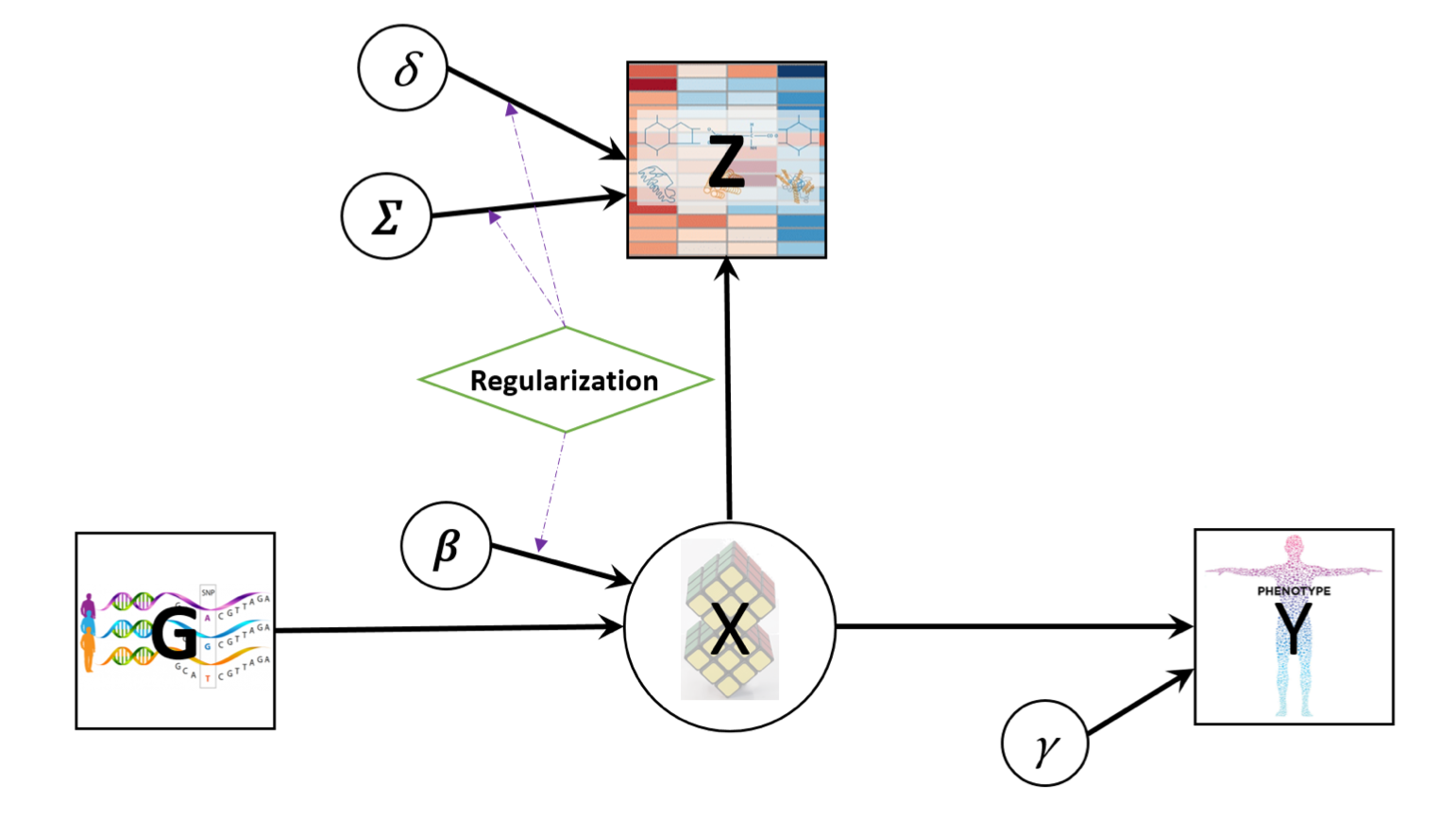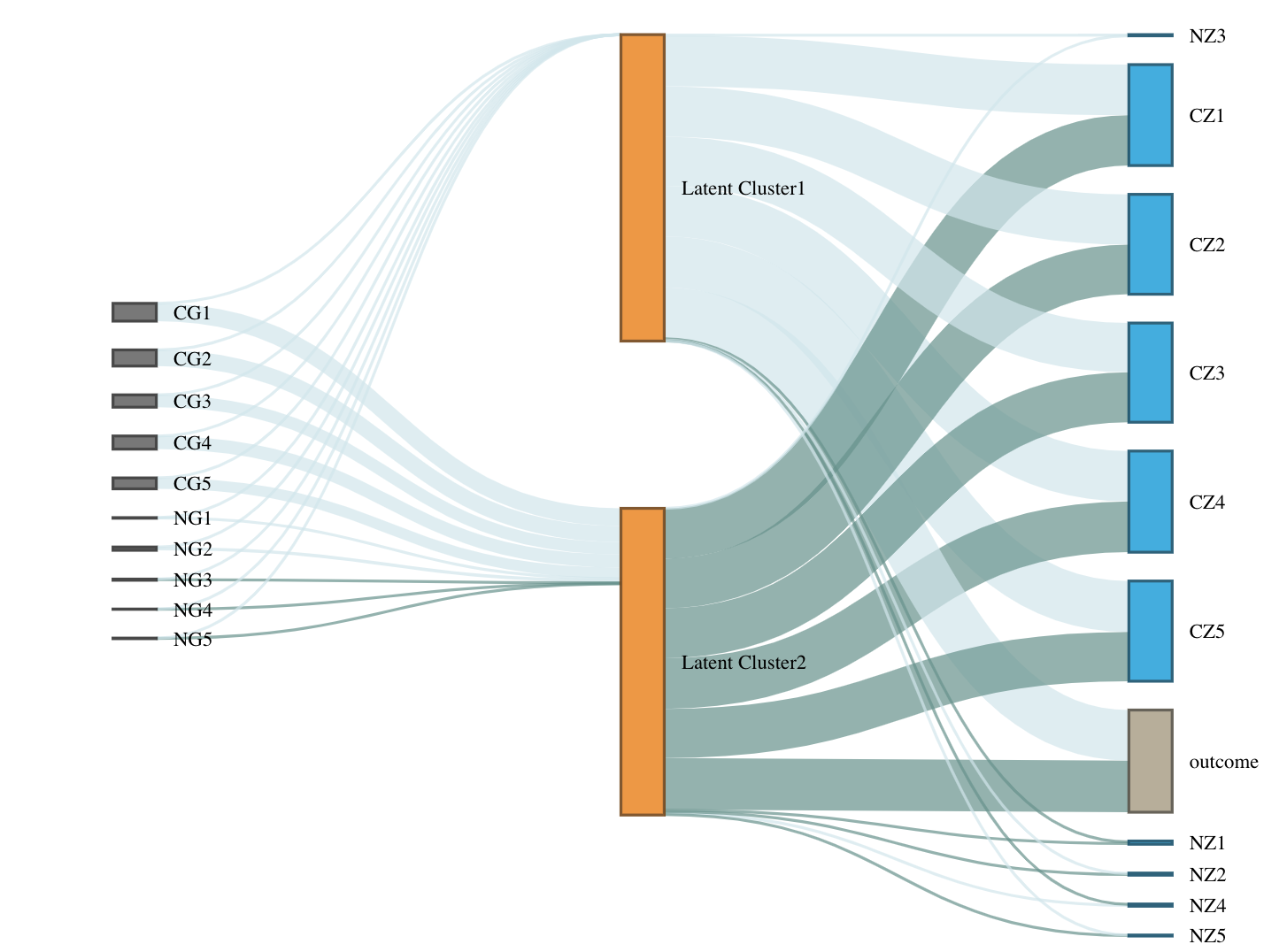# LUCIDus: Latent Unknown Clustering with Integrated Data

#### 2020-07-22

The LUCIDus package is aiming to provide researchers in the genetic epidemiology community with an integrative tool in R to obtain a joint estimation of latent or unknown clusters/subgroups with multi-omics data and phenotypic traits.

This package is an implementation for the novel statistical method proposed in the research paper “A Latent Unknown Clustering Integrating Multi-Omics Data (LUCID) with Phenotypic Traits1” published by the Bioinformatics. LUCID improves the subtype classification which leads to better diagnostics as well as prognostics and could be the potential solution for efficient targeted treatments and successful personalized medicine.

## Introduction to the LUCID (Latent Unknown Clustering with Integrated Data)

Multi-omics data combined with the phenotypic trait are integrated by jointly modeling their relationships through a latent cluster variable, which is illustrated by the directed acyclic graph (DAG) below. (A screenshot from LUCID paper)Let $$\mathbf{G}$$ be a $$n \times p$$ matrix with columns representing genetic features/environmental exposures, and rows being the observations; $$\mathbf{Z}$$ be a $$n \times m$$ matrix of standardized biomarkers and $$\mathbf{Y}$$ be a $$n$$-dimensional vector of disease outcome. By the DAG graph, it is further assumed that all three components above are linked by a categorical latent cluster variable $$\mathbf{X}$$ of $$K$$ classes and with the conditional independence implied by the DAG, the general joint likelihood of the LUCID model can be formalized into \begin{equation} \begin{aligned} l(\mathbf{\Theta}) & = \sum_{i = 1}^n\log f(\mathbf{Z}_i, Y_i|\mathbf{G_i}; \mathbf{\Theta}) \\ & = \sum_{i = 1}^n \log \sum_{j = 1}^K f(\mathbf{Z}_i|X_i = j; \mathbf{\Theta}_j) f(Y_i|X_i = j; \mathbf{\Theta}_j) f(X_i = j|\mathbf{G}_i; \mathbf{\Theta}_j) \end{aligned} \end{equation} where $$\mathbf{\Theta}$$ is a generic notation standing for parameters associated with each probability model. Additionally, we assume $$\mathbf{X}$$ follows a multinomial distribution conditioning on $$\mathbf{G}$$, $$\mathbf{Z}$$ follows a multivariate normal distribution conditioning on $$\mathbf{X}$$ and $$\mathbf{Y}$$ follows a normal/Bernoulli (depending on the specific data structure of disease outcome) distribution conditioning on $$\mathbf{X}$$. Therefore, the equation above can be finalized as \begin{equation} \begin{aligned} l(\mathbf{\Theta}) = \sum_{i = 1}^n \log \sum_{j = 1}^k S(\mathbf{G}_i; \boldsymbol{\beta}_j) \phi(\mathbf{Z}_i; \boldsymbol{\mu}_j, \boldsymbol{\Sigma}_j)f(Y_i;\mathbf{\Theta}_j) \end{aligned} \end{equation} where $$S$$ denotes the softmax function and $$\phi$$ denotes the probability density function (pdf) of the multivariate normal distribution.

To obtain the maximum likelihood estimates (MLE) of the model parameters, an EM algorithm is applied to handle the latent variable $$\mathbf{X}$$. Denote the observed data as $$\mathbf{D}$$, then the posterior probability of observation $$i$$ being assigned to latent cluster $$j$$ is expressed as \begin{equation} \begin{aligned} r_{ij} & = P(X_i = j|\mathbf{D}, \mathbf{\Theta}) \\ & = \frac{S(\mathbf{G}_i; \boldsymbol{\beta}_j) \phi(\mathbf{Z}_i; \boldsymbol{\mu}_j, \boldsymbol{\Sigma}_j)f(Y_i;\mathbf{\Theta}_j)}{\sum_{j = 1}^k S(\mathbf{G}_i; \boldsymbol{\beta}_j) \phi(\mathbf{Z}_i; \boldsymbol{\mu}_j, \boldsymbol{\Sigma}_j)f(Y_i;\mathbf{\Theta}_j)} \end{aligned} \end{equation} and the expectation of the complete log likelihood can be written as \begin{equation} \begin{aligned} Q(\mathbf{\Theta}) = \sum_{i = 1}^n\sum_{j = 1}^k r_{ij}\log\frac{S(\mathbf{G}_i; \boldsymbol{\beta}_j)}{r_{ij}} + \sum_{i = 1}^n\sum_{j = 1}^k r_{ij}\log\frac{\phi(\mathbf{Z}_i; \boldsymbol{\mu}_j), \boldsymbol{\Sigma}_j}{r_{ij}} + \sum_{i = 1}^n\sum_{j = 1}^k r_{ij}\log\frac{f(Y_i; \boldsymbol{\Theta}_j)}{r_{ij}} \end{aligned} \end{equation} At each iteration, in the E-step, compute the expectation of the complete data log likelihood by plugging in the posterior probability and then in the M-step, update the parameters by maximizing the expected complete likelihood function. Detailed derivations of the EM algorithm for LUDID can be found elsewhere.

# Framework to Fit the LUCID Model

The new version of LUCIDus package (ver 2.0.0) updates all the functions in the previous version and use Mclust function to initialize the algorithm to produce a more stable estimation. Although it is not backward compatible, it provides users with a more friendly model fitting framework and tables of estimates which are easy to interpret. The main functions in LUCIDus 2.0.0 are

Function Description
est.lucid() Estimate latent clusters using multi-omics data with/without the outcome of interest, and producing an lucid object; missing values in biomarker data (Z) are allowed
boot.lucid() Inference about the parameters of LUCID model based on bootstrap resampling method
summary() Summarize the results of LUCID model estimated by est.lucid(). It presents the results in a nice table with detailed explanation for parameters
plot() Use a Sankey diagram to visualize the LUCID model
predict() Predict the outcome based on a fitted LUCID model given new genetic data and biomarkers

Here, we use a simulated data set in the LUCID package to illustrate the framework of fitting LUCID model. The data contains 10 genetic features (5 causal, 5 non-causal), 10 biomarkers (5 causal, 5 non-causal), and a continuous outcome.

### Identify the number of latent clusters.

The first thing of fitting LUCID model is to identify the potential latent cluster number. Bayesian Information Criteria (BIC) is commonly used to compare and choose a group of non-nested models. Therefore we adapt the BIC to decide the number of latent clusters for LUCID. We can use tune.lucid() for this analysis.

> set.seed(1)
> tune.K <- tune.lucid(G = G2, Z = Z2, Y = Y2, K = 2:5)
> tune.K
$res.K K BIC 1 2 99025.54 2 3 99607.07 3 4 100196.34 4 5 100778.79$res.tune
NULL

$optimal Rho_G Rho_Z_InvCov Rho_Z_CovMu K BIC NA NA NA 2.00 99025.54  The function will return a list. list$res.K lists a series of models together with their BICs. list$optimal gives you the answer to the best K. Here, the model with K = 2 has the lowest BIC (99025.54) and hence we decide the optimal K to be 2. ### Fit the LUCID model We use est.lucid() function to fit the model. useY is an option to be taken good care of. By default, useY = TRUE, which means we’re interested in estimating the latent structure of the data and use the information of the outcome to cluster. On the other hand, if the primary question is to test the association of the cluster to outcome we should set it to FALSE since we do not want to bias the estimation of clusters by including the outcome in the estimation. Here is an example which focus on the estimation of the clustering. > fit1 <- est.lucid(G = G2, Z = Z2, Y = Y2, K = 2) initialize the LUCID ... iteration 1 : E-step finished. iteration 1 : M-step finished, loglike = -112022.6 iteration 2 : E-step finished. iteration 2 : M-step finished, loglike = -65392.77 iteration 3 : E-step finished. iteration 3 : M-step finished, loglike = -64538.53 iteration 4 : E-step finished. iteration 4 : M-step finished, loglike = -60593.42 iteration 5 : E-step finished. iteration 5 : M-step finished, loglike = -50891.01 iteration 6 : E-step finished. iteration 6 : M-step finished, loglike = -48932.31 iteration 7 : E-step finished. iteration 7 : M-step finished, loglike = -48932.31 Success: LUCID converges! > fit1 An object estimated by LUCID model Outcome type: normal Number of clusters: K = 2 Variance-Covariance structure for biomarkers: EII model Then use summary() to display the model parameters. > summary(fit1) ----------Summary of the LUCID model---------- K = 2 , log likelihood = -48932.31 , BIC = 99025.54 (1) Y (normal outcome): the mean and the sd of the Gaussian mixture Y for each latent cluster mu sd cluster1 -1.989017 0.9803328 cluster2 2.030915 1.0101779 (2) Z: estimates of biomarker means for each latent cluster cluster1 cluster2 CZ1 -2.007621361 2.016969684 CZ2 -1.963055183 1.940025207 CZ3 -2.003261127 2.006120325 CZ4 -2.006636548 1.984085152 CZ5 -1.998671158 1.965863805 NZ1 0.003405598 -0.028354380 NZ2 0.010965841 0.031840666 NZ3 -0.029590811 0.009409087 NZ4 0.018534552 0.005757923 NZ5 0.028345495 0.062087843 (3) E: the odds ratio of being assigned to each latent cluster for each exposure original OR CG1.cluster2 -0.70100547 0.4960863 CG2.cluster2 -0.72988086 0.4819664 CG3.cluster2 -0.72459572 0.4845204 CG4.cluster2 -0.64141179 0.5265485 CG5.cluster2 -0.68018375 0.5065239 NG1.cluster2 -0.21877995 0.8034985 NG2.cluster2 0.08447478 1.0881454 NG3.cluster2 -0.04780871 0.9533161 NG4.cluster2 0.01291939 1.0130032 NG5.cluster2 0.01306431 1.0131500 For a better understanding of the model, we can visualize the LUCID model by plot(). plot(fit1) knitr::include_graphics("fig3.png")### Variable Selection Procedure As we can see from the table and the Sankey diagram, many genetic effects and biomarker effects are almost 0. To follow the principle of parsimony, it’s reasonable to conduct a variable selection procedure to achieve a simpler model. There are 3 tuning parameters in the variable selection. Rho_G is the penalty for genetic features and Rho_InvCov and Rho_CovMu are the penalties for biomarkers. To choose the best combination of tuning parameters, we perform a grid search and use BIC to identify the optimal choice. Here is an example of search 18 combinations. > tune.par <- tune.lucid(G = G2, Z = Z2, Y = Y2, family = "normal", K = 2, + Rho_G = c(0.01, 0.02), + Rho_Z_InvCov =c(0.1, 0.15, 0.2), + Rho_Z_CovMu = seq(80, 100, by = 10)) > tune.par$res.K
K
1 2

$res.tune Rho_G Rho_Z_InvCov Rho_Z_CovMu K BIC 1 0.01 0.10 80 2 98958.38 2 0.01 0.10 90 2 98657.88 3 0.01 0.10 100 2 108764.74 4 0.01 0.15 80 2 99103.11 5 0.01 0.15 90 2 98802.57 6 0.01 0.15 100 2 98678.07 7 0.01 0.20 80 2 99289.15 8 0.01 0.20 90 2 98988.58 9 0.01 0.20 100 2 98864.04 10 0.02 0.10 80 2 98983.32 11 0.02 0.10 90 2 98682.81 12 0.02 0.10 100 2 98558.35 13 0.02 0.15 80 2 99128.04 14 0.02 0.15 90 2 98827.50 15 0.02 0.15 100 2 98703.01 16 0.02 0.20 80 2 99314.08 17 0.02 0.20 90 2 99013.51 18 0.02 0.20 100 2 98888.97$optimal
Rho_G Rho_Z_InvCov Rho_Z_CovMu K      BIC
12  0.02          0.1         100 2 98558.35

The best combination is Rho_G = 0.02, Rho_Z_InvCov = 0.1 and Rho_Z_CovMu = 100. Next, refit the model with penalties.

> fit2 <- est.lucid(G = G2, Z = Z2, Y = Y2, K = 2, tune = def.tune(Rho_G = 0.02, Rho_Z_InvCov = 0.1, Rho_Z_CovMu = 1000, Select_G = TRUE, Select_Z = TRUE))
initialize the LUCID ...
iteration 1 : E-step finished.
iteration 1 : M-step finished,  loglike =  -59892.22
iteration 2 : E-step finished.
iteration 2 : M-step finished,  loglike =  -49756.34
iteration 3 : E-step finished.
iteration 3 : M-step finished,  loglike =  -49750.85
iteration 4 : E-step finished.
iteration 4 : M-step finished,  loglike =  -49750.85
Success: LUCID converges! 

Let’s check the variables selected by LUCID.

> fit2$select$selectG
CG1   CG2   CG3   CG4   CG5   NG1   NG2   NG3   NG4   NG5
TRUE  TRUE  TRUE  TRUE  TRUE  TRUE FALSE FALSE FALSE FALSE

\$selectZ
CZ1   CZ2   CZ3   CZ4   CZ5   NZ1   NZ2   NZ3   NZ4   NZ5
TRUE  TRUE  TRUE  TRUE  TRUE FALSE FALSE FALSE FALSE FALSE 

We can then refit the model by using the selected features.

### Inference on Parameters

It’s hard to derive the asymptotic distribution of the estimates of LUCID. Thus we use a bootstrap method to do inference on parameters. It is realized by the function boot.lucid. Let’s try bootstrap on model 1.

> boot <- boot.lucid(G = G2, Z = Z2, Y = Y2, model = fit2, R = 100)
initialize the LUCID ...
iteration 1 : E-step finished.
iteration 1 : M-step finished,  loglike =  -63133.32
iteration 2 : E-step finished.
iteration 2 : M-step finished,  loglike =  -48932.81
iteration 3 : E-step finished.
iteration 3 : M-step finished,  loglike =  -48932.31
iteration 4 : E-step finished.
iteration 4 : M-step finished,  loglike =  -48932.31
Success: LUCID converges! 

We can add bootstrap SE and 95% CI to the summary table by specifying the option se.

summary(fit1, boot.se = boot)

## Acknowledgments

• Cheng Peng, Ph.D.
• Zhao Yang, Ph.D.
• USC IMAGE Group2

1. Supported by the National Cancer Institute at the National Institutes of Health Grant P01 CA196569↩︎